International Journal of Mechanical Engineering and Applications
Volume 4, Issue 6, December 2016, Pages: 242-248

Applicability of the Hertz Contact Theory to Rolling Rubber Cylinders Model

Chu Hongyan, An Ran, Cai Ligang

Mechanical Engineering, Beijing University of Technology, Beijing, China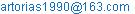(An Ran)

Chu Hongyan, An Ran, Cai Ligang. Applicability of the Hertz Contact Theory to Rolling Rubber Cylinders Model. International Journal of Mechanical Engineering and Applications. Vol. 4, No. 6, 2016, pp. 242-248. doi: 10.11648/j.ijmea.20160406.15

Received: October 20, 2016; Accepted: December 7, 2016; Published: December 28, 2016

Abstract: Hertz contact theory (HCT) is a study of contact stress and deformation which is widely used in many engineering fields. However, there are many limiting conditions for the applications of the HCT, in this paper, the contact model between a plastic cylinder and a rubber cylinder does not fit two limiting conditions: Small deformation and no rigid body motion. By applying three-dimensional finite element models, the deformation and the maximum contact stress of two rotating cylinders have been analyzed. Comparing the simulation results and the theoretical results, the applicability of Hertz contact theory to this model has been discussed. The results show that: In the condition of large deformation, the theoretical results of maximum contact stress fit the actual situation, but the half-width of the contact area does not fit. Under the rolling condition, the theoretical maximum contact stress has a large deviation to simulation result.

Keywords: Hertz Contact Theory, Large Deformation, Contact Stress, Elastic Cylinder

1. Introduction

Hertz contact theory is a classical contact theory which can calculate the contact stress and deformation. Since the result of hertz contact theory is an approximate solution in the context of many assumptions, it is less suitable in many fields. In recent years, with the continuous development of finite element analysis software, it is also a effective way to analysis the contact problems using finite element analysis software than using Hertz contact theory to calculate, however, to analysis the contact model, a accurate model and suitable constraints are needed, and it is difficult to built a accurate model. The model of two elastic cylinders is widespread in imprinter inking system and other fields, but it is difficult to analysis the contact stress and the deformation with finite element analysis software. Using hertz contact theory to calculate the contact stress and the deformation can save a lot of time. in this paper, the author use ANSYS to analyze the model of two rolling cylinders, and analyze and compare the value of contact stress and the deformation to discuss the applicability of Hertz contact theory to this contact model, to provide some guidance to some engineering fields.

2. Hertz Contact Theory

The Hertz contact theory is published in 1882 by Heinrich Hertz, he solved the contact problem of two elastic bodies with curved surfaces. This still-relevant classical solution provides a foundation for modern problems in contact theory hertz contact theory is widely used in many engineering fields. However, there are many limiting conditions for the applications of the HCT, there are some assumptions when use the Hertz contact theory to calculate the contact stress and the deformation: (1) both are elastic bodies; (2) no rigid body motion; (3) small deformation in contact area; (4) the significant dimensions of the contact area should be much smaller than the dimensions and the radii of curvature of the bodies in contact. When the above conditions are satisfied, using hertz contact theory to calculate the contact stress and strain is pretty accurate. But in practice, it is possible to obtain a accurate calculation, even if it does not satisfy some assumptions. By using HCT, Junjun Ding in Xi'an Jiaotong University analyzes the rail-wheel contact problems, the values of Calculated result and simulation result are very similar . Mingyong Liu in Chongqing University has a research on thermo elastic hydrodynamic lubrication based on finite line contact, he obtains the distribution of the pressure in contact area . Zongde Fang in Northwestern Polytechnical University analyzed a pair of spiral bevel gear, discussed the applicability of Hertz contact theory to gear contact model. . This paper will discuss the contact stress and the deformation of two different rolling cylinders. one of the cylinder is made of plastic, the surface of another cylinder is made of rubber. due to the small elastic modulus of rubber, it will have a big deformation in contact area, and the cylinders are rolling, so it does not fit the assumptions of no rigid body motion and small deformation. Figure 1 shows a typical example of Hertz contact.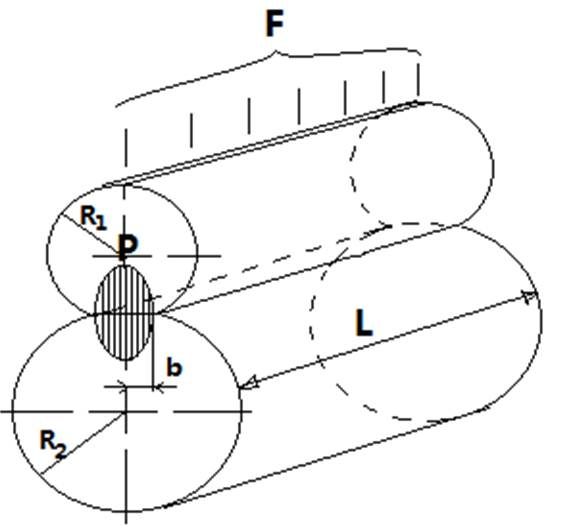Figure 1. Typical example of Hertz contact.

In this paper, Author will analyze the contact stress and deformation of two different cylinders, which the hard one is made up by plastic, the soft one is rubber. Because the soft one has a rubber surface, due to the Elastic Modulus of rubber is much smaller than plastic, it will have a large deformation in rubber cylinder, this condition does not fit the assumption of small deformation in contact area. And when the cylinders start to rolling, this model does not fit the assumption of no rigid body motion. Author will discuss the Applicability of the Hertz contact theory to these two conditions.

The Figure 2 shows that two cylinders can be replaced by one equivalent cylinder and a rigid planar, the radius of two cylinders are R1 and R2 , if: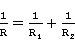(1)

The R is the radius of equivalent cylinder which is called equivalent curvature radius.

The Elastic Modulus of two cylinders are E1 and E2, and the Poisson ratios are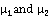, if: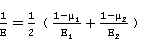(2)

E can be called the equivalent elastic modulus.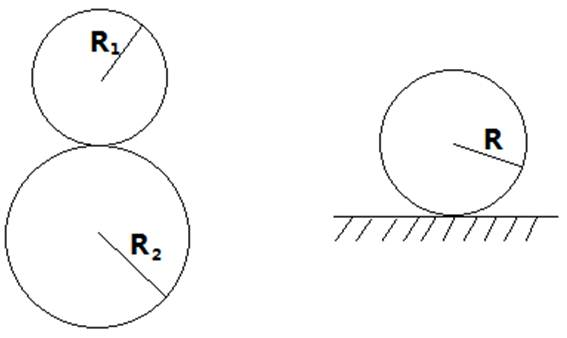Figure 2. Equivalent cylindrical and equivalent flat.

Under the unit length load w, two cylinders Squeeze each other, and the contact area is a narrow rectangle, according to Hertz contact theory, we can get a simplified contact model [6-9] shown in Figure 3.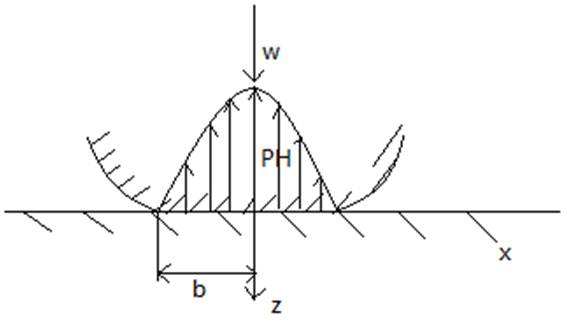Figure 3. Simplified model.

The half-width of area can be expressed as b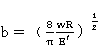(3)

P is the contact stress on the contact area. If two elastic nonconforming bodies contact together, then, according to the HCT, The distribution of the contact pressure in this elliptical area represents a semi-ellipsoid, which can be expressed as [10-11]: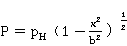(4)

The maximum contact stress can be calculated by formula 5.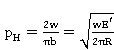(5)

b is the value of half width of the contact area,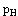is the maximum contact stress.

3. Finite Element Model

3.1. ANSYS Mechanical Software

ANSYS Mechanical is a finite element analysis tool for structural analysis, including linear, nonlinear and dynamic studies. This computer simulation product provides finite elements to model behavior, and supports material models and equation solvers for a wide range of mechanical design problems. ANSYS Mechanical also includes thermal analysis and coupled-physics capabilities involving acoustics, piezoelectric, thermal–structural and thermo-electric analysis.

3.2. Units and Contact Types Selection

The contact model is made up by two cylinders, the hard cylinder is made of plastic, the soft one has a rubber surface, this contact model of two elastic cylinders is widespread in imprinter inking system and other fields, due to the Elastic Modulus of rubber is much smaller than plastic, there is a large deformation in soft cylinder. The soft cylinder has a steel shaft and a rubber surface, the center steel shaft has a very small deformation when contacting with the hard cylinder. therefore, we establish the rubber surface of soft cylinder only, solid186 is a Three-dimensional 20-node unit which used to applies to analog irregular grid and simulate the incompressible elastic material. Mooney-Rivlin model is suitable for simulating large deformation of super-elastic material and simulate most of mechanical properties of rubber. In this paper, the author selected Solid 186 unit and Mooney-Rivlin model to simulate the rubber of soft cylinder, MPC 184 unit is a connecting unit used to simulate the soft cylinder rotating about its center. The type of contact should be selected when creating contact model. The type of contact problems can be divided into two basic types: rigid – flexible type and flexible – flexible type, since the large Elastic Modulus gap of cylinder’s material, we selected a rigid - flexible contact in this model.

3.3. The Establishment of Finite Element Model of Two Cylinders

Defined unit type and material parameters of two cylinders, Elastic modulus of the plastic cylinder is 4000MPa, Poisson's ratio is 0.33, for the diameter is 65mm, and the axial length of cylinder is 30mm; Modeling the rubber part of soft cylinder, Setting the elastic modulus of the rubber is 1.16MPa. The parameter of C10 is 0.27 and the C01 is 0.027 in Mooney-Rivlin model. The outer diameter of soft cylinder is 65mm, and the inner diameter is 45mm. the axial length of cylinder is 30mm. Using the swept meshing to mesh the model. As shown in Figure 4, the soft cylinder is tangent to the hard cylinder but without extruding.

Since the size of contact area is much smaller than the whole size of the cylinder, a sufficient density of mesh in contact area is needed. In order to ensure the precision of finite element analysis result and reduce the system resource, the contact area is estimated and fine meshed. so, we refine the mesh of the soft cylinder in contact area to provide an accurate enough contact model.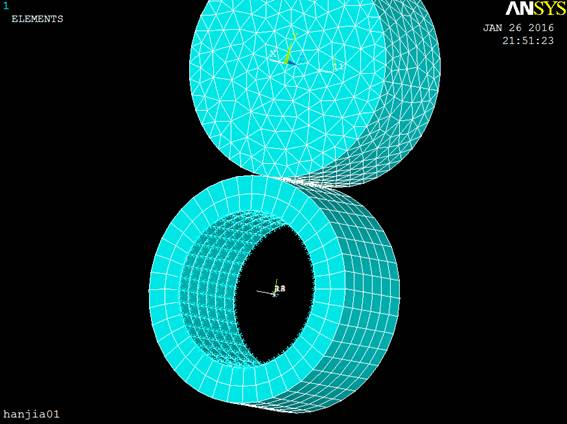Figure 4. Soft and hard cylinder model.

4. Finite Element Model Simulation and Theoretical Calculation

4.1. Pure Extrusion Model

In this part, under the force of the load in hard cylinder, two cylinders start to contact in contact area. Select the center points at both ends of the hard cylinder, exerts a downward forces on these two point to keep the two cylinders are in a compressive state, these two ramp load forces will finish the loading in 1 second, At the same time we must constraint the displacement of X, Z direction and rotation of X, Y, Z directions of two points to make sure it can only be moved in the Y direction. the constraint of these node is shown in Fig. 5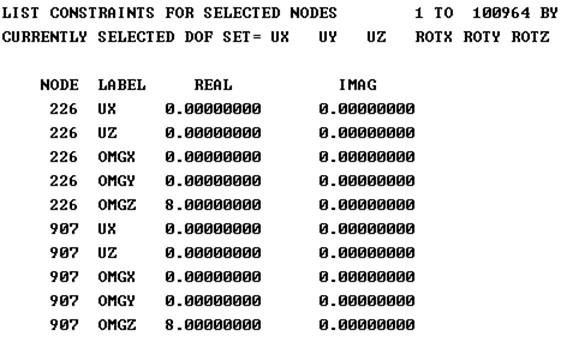Figure 5. The constraint of displacement.

4.1.2. Finite Element Simulation Results

Setting the forces of load is 100N, extracted the contact press of contact area as shown as Fig. 6.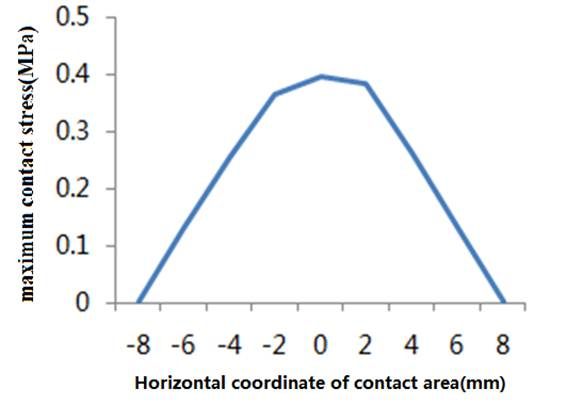Figure 6. Contact stress of Soft cylindrical.

Figure 6 shows that the center of contact area has the maximum contact stress in contact area. The maximum value is 0.397MPa, the width of the contact area is about 16.38mm, the contact stress and the deformation in contact area is showed in Figure 7 and 8, the obvious deformation occurred in soft cylinder, The maximum deformation in Y direction is 1.604mm.Figure 7. Contact stress schematic.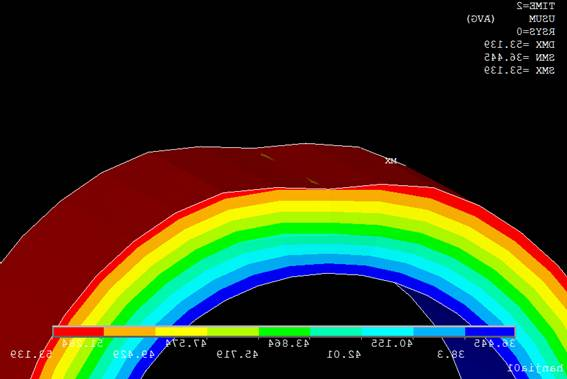Figure 8. Deformation schematic.

4.2. Squeezing Rolling Model of Two Cylinders

On the basis of pure squeezing model, adding the rolling of the hard cylinder, the hard cylinder around its geometric center in circumferential direction, the rotational speed is 8rad/s, and the rotation time is 1s, under the driven of frictionally, the soft cylinder start to circumferentially rotate.

4.2.2. Finite Element Simulation Results

In this part, the author will discuss the Applicability of the Hertz contact theory to the rolling model. Setting the force of load is 100N, picking the node 4407 as shown as in Fig. 9.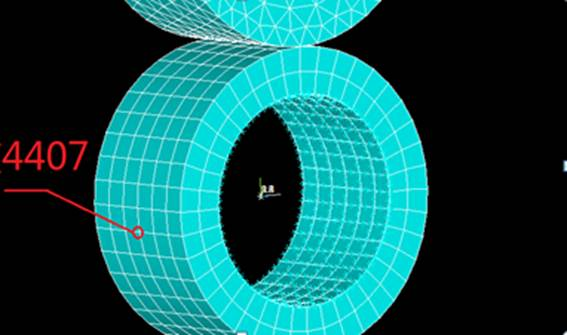Figure 9. Pick the node.

Extract the whole changing process of the contact stress of node 4407, in Fig 10 we can see the curve of contact stress.

Whit the node goes into the contact area, the contact stress begin to increase, and then slightly decreased, and then it increase again, in middle contact area, the contact stress reached the maximum valve.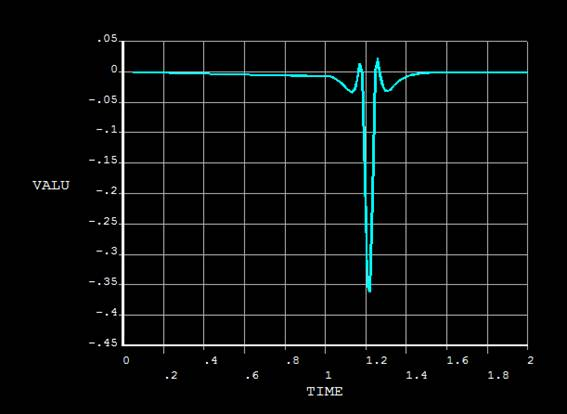Figure 10. Contact stress of node.

The contact stress on contact area is shown in Fig 11,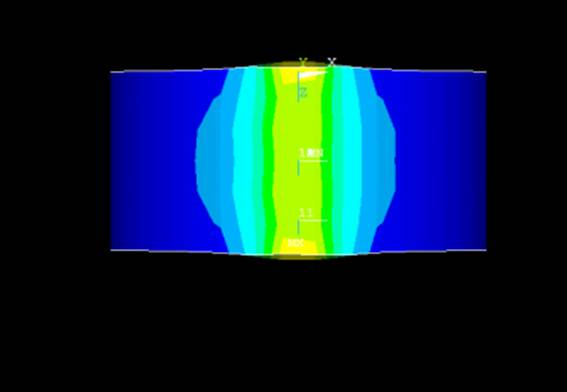Figure 11. Contact stress in contact area.

As shown in Fig. 10 The maximum contact stress of node 4407 in the whole rolling process is 0.361MPa.

Extract the Y-direction displacement of node 4407, as shown in Fig. 12.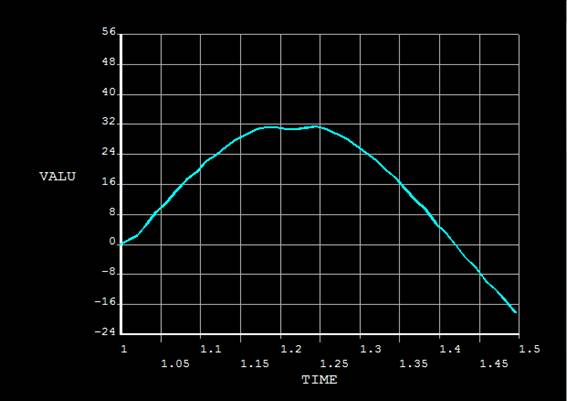Figure 12. Y direction displacement.

The radius of the soft cylinder is 32.5mm, due to the squeezing of the hard cylinder, the node has a displacement in y-direction. After a simple calculation, we can know that the maximum deformation of node 4407 is 1.691mm. from Fig. 13 we can clearly see that there is big deformation in contact area in soft cylinder.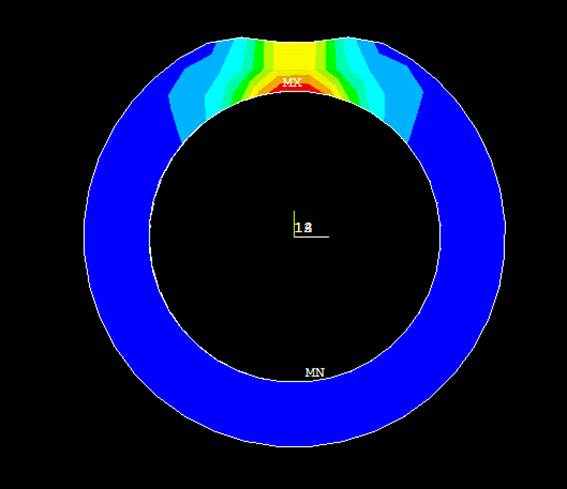Figure 13. Deformation in soft cylinder.

4.3. Theoretical Calculations of Hertz Contact Theory

Using the hertz contact theory to calculate the contact stress of the pure squeezing cylinders model, By using the formula (1), (2) and (5), we can calculate the equivalent elastic modulus, equivalent curvature radius and contact stress, E=2.319MPa, R=16.25mm,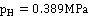. That is, when squeezing only, the maximum contact stress is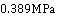. By the formula (3), we can calculate the half-width of contact area, b = 10.906mm.

5. The Applicability of Hertz Contact Theory to the Model

5.1. The Applicability of Hertz Contact Theory to the Situation of Large Deformation

There is a large deformation of soft cylinder in contact area when squeezing with the hard cylinder. Thus, the assumptions of small deformation does not fit this model, but the applicability of Hertz contact theory to the maximum contact stress of this model is still needs to have a further study. Considering the situation of large deformation only, the results of maximum contact stress is quite similar. The simulation result of finite element model shows that the maximum contact stress in the contact area is 0.397MPa, and the theoretical results of Hertz contact theory is 0.389MPa, these two values are very close, but there is still a little difference, The error between the two values is: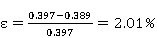(6)

Although the assumptions of small deformation is not suitable for this model, the values of maximum contact stress are still very close, that is, under the condition of large deformation in contact area, using the Hertz contact theory to calculate the maximum contact stress is still accurately. According to the result of the simulation result of finite element model, the half-width of contact area is 8.19mm, and the value of theoretical result of half-width is 10.90mm, The error between the two values is: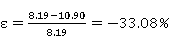(7)

There is a big gap between the theoretical results of hertz contact theory and the simulation result in the half-width of contact area, due to the small Elastic Modulus of rubber, the soft cylinder has a large deformation in contact area, this does not fit the assumption of hertz contact theory. therefore, when calculating the half-width of contact area, using hertz contact theory is improper.

In Hertz contact theory, the distribution of contact stress in contact area can be described by formula (4), compared with the simulation results, we can see that the maximum contact stress is quite similar, as shown in Fig. 14.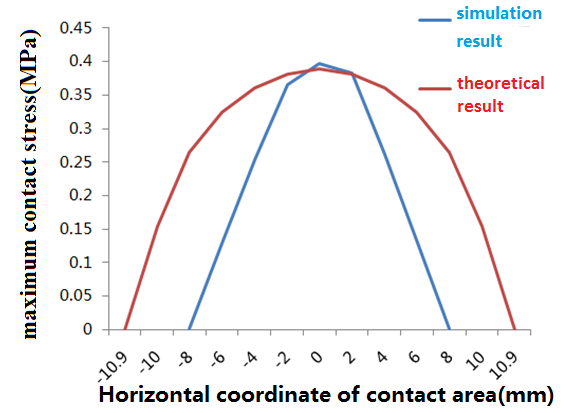Figure 14. The distribution of contact stress.

Figure 14 shows that the maximum contact stress of simulation result and theoretical result are very close, and both of them are in the middle of contact area.

The half-width of the contact area and the distribution of contact stress is quite different. The half-width of contact area of simulation result is narrower than theoretical result.

5.2. The Applicability of Hertz Contact Theory to the Rolling Model

In the hertz contact theory, the assumptions of no rigid body motion does not fit this model. From the simulation result we can know that the maximum contact stress in contact area is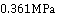and the theoretical result of maximum contact stress is 0.389MPa, the error between them is: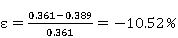(8)

From formula (8) we know that there will be a large error when calculating the maximum contact stress of contact area in this rolling model. That is, under the condition of rolling model, the theoretical result of the hertz contact theory does no accurate.

In order to analyze the trends of error, comparing the maximum contact stress of simulation result and calculation result under different loads.

When the cylinders start to rolling, there is a big gap between the simulation value and the theoretical value, In order to analyze the variation tendency of the error, we compared the value of contact stress and the deformation under different loads, The results obtained are shown in Figure 15.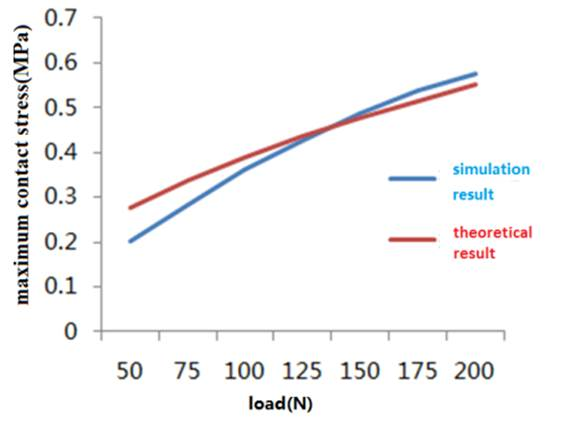Figure 15. Maximum contact stress.

From figure 15 we can see hertz contact theory does not fit the rolling model, it has a large error when calculating the maximum contact stress, and the calculation result is bigger than simulation result when the load is small, smaller than simulation result when the load is large.

6. Conclusion

Hertz contact theory is a classical contact theory which can calculate the contact stress and deformation, but there are many limiting conditions for the applications of the HCT: (1) both are elastic bodies;(2) no rigid body motion;(3) small deformation in contact area;(4) the significant dimensions of the contact area should be much smaller than the dimensions and the radii of curvature of the bodies in contact. By applying three-dimensional finite element models, the deformation and the maximum contact stress of two rotating cylinders have been analyzed. Compared the simulation results and the theoretical results, the applicability of Hertz contact theory to this model has been discussed. The results showed that:1. In the condition of large deformation in contact area, the values of maximum contact stress of simulation result and the theoretical result are very close, but there is a big gap between the theoretical results of hertz contact theory and the simulation result in the half-width of contact area; 2. Hertz contact theory does not apply to rolling model when calculating the maximum contact stress.

Acknowledgements

First of all, I would like to extend my sincere gratitude to my Hierophant, Chu Hongyan, for her instructive advice and useful suggestions on my thesis. I am deeply grateful of her help in the completion of this thesis.

Supported by National Natural Science Foundation (51675010), Science and technology project of Beijing Municipal Education Commission (KM201710005015)

References

1. Tian Hongliang, Zhu Dalin, Fang Zifan, Qin Hongling. Hertz contact for 129 years [J]. Journal of China Three Gorges University (NATURAL SCIENCE EDITION), 2011, 06: 61-71. J. Clerk Maxwell, A Treatise on Electricity and Magnetism, 3rd ed., vol. 2. Oxford: Clarendon, 1892, pp.68–73.
2. Sun Shulei, Ding Junjun, Li Fei, Huang Yunhua, Zhou Zhang Yi. Research on the wheel / rail contact problem of metro vehicles [J]. urban rail transit research, 2013, 08: 39-43.
3. Mingyong Liu. Study on thermo elastic hydrodynamic lubrication of helical gears based on finite line contact [D]. Chongqing University, 2013
4. Wang Zubing, Fang Zongde, Su Jin. Study on meshing characteristics of profile modification spur gear [J]; mechanical transmission; 12 phase of 2011.
5. S.-H. Gao, G. Meng, X.-H. Long. Study of milling stability with Hertz contact stiffness of ball bearings [J]. Archive of Applied Mechanics, 2011, 818.
6. Ma Wen. Calculation and finite element analysis of rolling bearing contact problem [J]. mechanical engineering and automation, 2011, 06: 51-53.
7. W. Yan, F. D. Fischer. Applicability of the Hertz contact theory to rail-wheel contact problems[J]. Archive of Applied Mechanics,2000,704.
8. V. G. Vil’ke, I. I. Kosenko, E. B. Aleksandrov. On computer implementation of the Hertz elastic contact model and its simplifications [J]. Regular and Chaotic Dynamics, 2009, 146.
9. Xu Kai, Su Jianxin, Zhou Yongdan, Qiu Ming, Wang Weisheng. Finite element analysis of line contact and point contact of gears [J]. mechanical transmission, 2014, 08: 77-81.
10. Xiaoyong Li, Shilong Wang, Jie Zhou. Analysis of elliptical Hertz contact of steel wires of stranded-wire helical spring [J]. Journal of Mechanical Science and Technology, 2014, 287.
11. Wan Fei, Li Guo-xi, Gong Jing-zhong, Wu Bao-zhong. Dynamic study of anti-backlash gears and angle-contact ball bearing based on a simplification of the Hertz contact theory [J]. Proceedings of the Institution of Mechanical Engineers, 2016, 2301.

 Contents 1. 2. 3. 3.1. 3.2. 3.3. 4. 4.1. 4.2. 4.3. 5. 5.1. 5.2. 6.
Article ToolsAbstractPDF(506K)